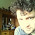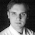## Tuesday, August 16, 2011

### ttrTests Experimentation

I was intrigued by the CRAN update on a package ttrTests, especially since quantstrat is not built for backtesting system parameters (not true since quantstrat offers parameter testing and I will demonstrate in later post) and analyzing system performance as I mentioned in A Quantstrat to Build On Part 6.  ttrTests offers a nice start to my ideal setup for system development, testing, and reporting.  In upcoming posts, I hope to build some functionality on top of ttrTests to accomplish my objectives.

I proposed a basic counting system in A Quantstrat to Build On Part 6, but randomly assigned a 50 week (250 days or 1 trading year) parameter to the count.  With the help of ttrTests and a couple of index series from Yahoo!Finance, let’s see what parameters work best.  Then we can aggregate and graph to visualize the results.  As always, THIS IS NOT INVESTMENT ADVICE, and I welcome comments and suggestions.

`#let's define our silly countupdown function#as a sample of a custom ttr ruleCUD <- function(x,params=50,...) {	#CUD takes the n-period sum of 1 (up days) and -1 (down days)	temp <- ifelse(runSum(ifelse(ROC(x,1,type="discrete") > 0,1,-1),params)>=0,1,0)	#replace NA with 0 at beginning of period	temp[is.na(temp)] <- 0	temp}   require(ttrTests)require(quantmod)require(lattice)require(reshape2)require(PerformanceAnalytics)   #defaults functions is overridden by ggplot2 and plyr if loaded#and will cause problems if you want to use ttrTests concurrently   tckrs <- c("GSPC","RUT","N225","GDAXI","DJUBS")   for (i in 1:length(tckrs)) {	getSymbols(paste("^",tckrs[i],sep=""),from="1896-01-01",to=Sys.Date())	test_price <- as.vector(get(tckrs[i])[,4])	#do parameter tests but plot=FALSE	#we will plot later	#if you want plot=TRUE make sure you add dev.new() here	param_results <- paramStats(x=test_price, ttr = CUD, start = 20, nSteps = 30, stepSize = 10,		restrict = FALSE, burn = 0, short = FALSE, condition = NULL,		silent = TRUE, TC = 0.001, loud = TRUE, plot = FALSE, alpha = 0.025,		begin = 1, percent = 1, file = "", benchmark = "hold")	#get excess returns and add to matrix	ifelse(i==1,param_all <- param_results[],		param_all <- cbind(param_all,param_results[]))	#get best parameter and add to matrix	ifelse(i==1,param_best <- param_results[],		param_best <- rbind(param_best,param_results[]))}rownames(param_best) <- tckrsprint(param_best)   param_all <- cbind(param_results[],param_all)#fix rownames and colnames for param_allcolnames(param_all) <- c("parameters",tckrs)   df <- as.data.frame(param_all)df.melt <- melt(df,id.vars=1)colnames(df.melt) <- c("parameters","index","excessreturn")param_plot <- xyplot(excessreturn~parameters,group=index,data=df.melt,	auto.key=TRUE,type="l",main="Excess Returns by Parameter")#jpeg(filename="excess return by parameter.jpg",	quality=100,width=6.25, height = 6.25,  units="in",res=96)print(param_plot)#want to add points for max but unsure how currently#df.melt[which(df.melt\$parameters==param_best[1,] & df.melt\$index==rownames(param_best) ),3]dev.off()   #get performance summary for the best parametersfor (i in 1:length(tckrs)) {	dev.new()	#jpeg(filename=paste(tckrs[i],"performance summary.jpg",sep=""),	#	quality=100,width=6.25, height = 6.25,  units="in",res=96)	ret <- merge(lag(CUD(get(tckrs[i])[,4],		coredata(param_best),k=1))*ROC(get(tckrs[i])[,4],type="discrete", n=1),		ROC(get(tckrs[i])[,4],type="discrete", n=1))	colnames(ret)<-c(paste(tckrs[i]," CUD System",sep=""),tckrs[i])	charts.PerformanceSummary(ret,ylog=TRUE)	#dev.off()	ret[1,]<-0	price_system <- merge(get(tckrs[i])[,4],		lag(CUD(get(tckrs[i])[,4],		coredata(param_best),k=1))*get(tckrs[i])[,4],		cumprod(1+ret[,1])*coredata(get(tckrs[i]))[coredata(param_best),4])	price_system[which(price_system[,2]==0),2] <- NA	colnames(price_system) <- c("Out","In","System")   dev.new()	#jpeg(filename=paste(tckrs[i],"entry analysis.jpg",sep=""),	#	quality=100,width=6.25, height = 6.25,  units="in",res=96)	chartSeries(price_system\$System,theme="white",log=TRUE,up.col="black",		yrange=c(min(price_system[,c(1,3)]),max(price_system[,c(1,3)])),		TA="addTA(price_system\$Out,on=1,col=2);		addTA(price_system\$In,on=1,col=3)",		name=paste(tckrs[i]," Linear Model System",sep=""))	#dev.off()}`

Created by Pretty R at inside-R.org

1.Your N225 linear model graph really drives home the point that in/out systems do very well when there are large, sustained movements in either direction. Most simple moving average based strategies during 2008/2009 avoid losses of 20% or more, but then capitalize on the 30% gains. Similarly most MA strategies would have been short the Dow around 12,300 in July 2011.

2.ttrTests isn't likely to see a lot of love, as the author has moved on to other things.

I'd really like to port those tests into quantstrat, but the -1,0,1 based function model is really painful (impossible) for strategies that are more complex in their rules. I think we'd need to port the *metrics*, but adapt for transactions from blotter, and possibly borrow a page from Pat Burns' random trades literature to get something similar to the Monte Carlo simulation David does in ttrTests.

Also, your comment about quantstrat not being set up for parameter testing isn't true, see demo scripts parameterTest.R and parameterTestMACD.R

We hope to polish that parameter testing functionality up over coming months, possibly adding some objective function based parameter optimization, or 'in-sample' training periods.

3.I will strike through the absence of parameter testing in quantstrat since I completely forgot about that very helpful addition. I hope to provide an example to complement the documentation.

Let me know if I can help. I love the bootstrap tests and easy buy-hold comparison of ttrTests, but there are a lot of missing elements. I have not officially contributed to any r projects but would love to contribute in whatever way possible.

4.We're always looking for additional contributors. Please email or call me to discuss.

Cheers, Brian